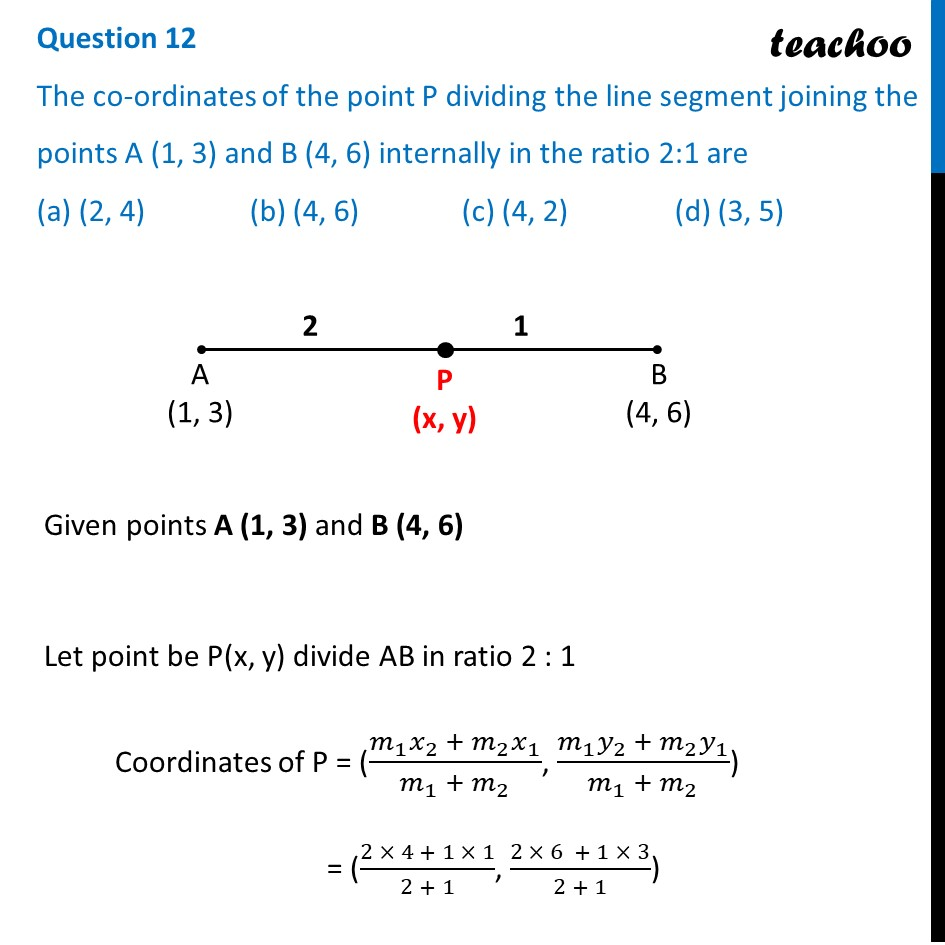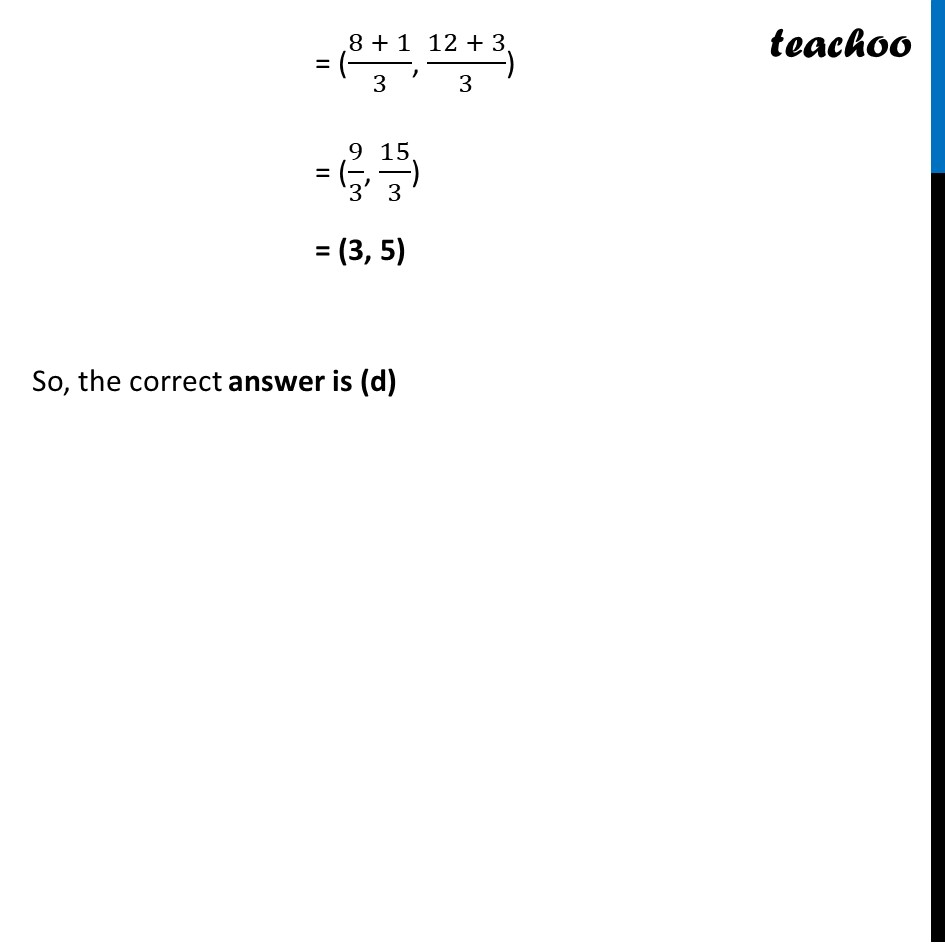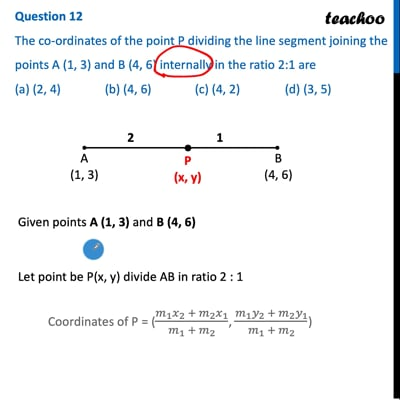CBSE Class 10 Sample Paper for 2022 Boards - Maths Basic [MCQ]

Class 10
Solutions of Sample Papers for Class 10 Boards

## (a) (2, 4)   (b) (4, 6)   (c) (4, 2)   (d) (3, 5)This video is only available for Teachoo black users

Solve all your doubts with Teachoo Black (new monthly pack available now!)

### Transcript

Question 12 The co-ordinates of the point P dividing the line segment joining the points A (1, 3) and B (4, 6) internally in the ratio 2:1 are (a) (2, 4) (b) (4, 6) (c) (4, 2) (d) (3, 5) Given points A (1, 3) and B (4, 6) Let point be P(x, y) divide AB in ratio 2 : 1 Coordinates of P = ((𝑚_1 𝑥_2 + 𝑚_2 𝑥_1)/(𝑚_1 + 𝑚_2 ), (𝑚_1 𝑦_2 + 𝑚_2 𝑦_1)/(𝑚_1 + 𝑚_2 )) = ((2 × 4 + 1 × 1)/(2 + 1), (2 × 6 + 1 × 3)/(2 + 1)) = ((8 + 1)/3, (12 + 3)/3) = (9/3, 15/3) = (3, 5) So, the correct answer is (d)